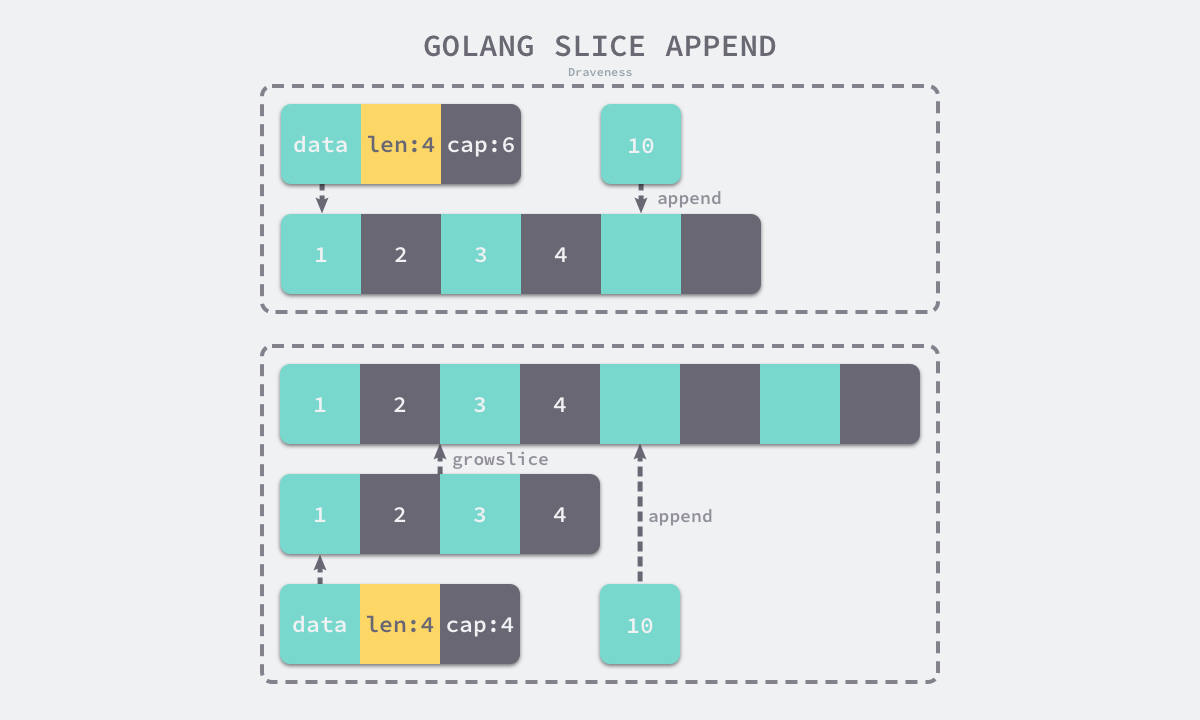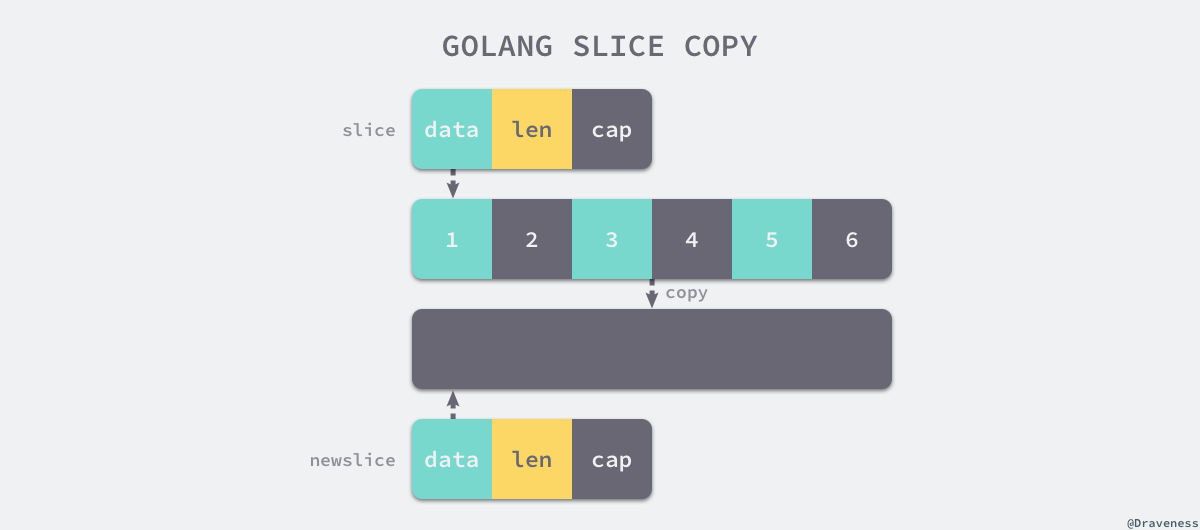Go 语言切片的实现原理

# 3.2 切片 #

[]int
[]interface{}


func NewSlice(elem *Type) *Type {
if t := elem.Cache.slice; t != nil {
if t.Elem() != elem {
Fatalf("elem mismatch")
}
return t
}

t := New(TSLICE)
t.Extra = Slice{Elem: elem}
elem.Cache.slice = t
return t
}


## 3.2.1 数据结构 #

• Data 是指向数组的指针;
• Len 是当前切片的长度；
• Cap 是当前切片的容量，即 Data 数组的大小：
type SliceHeader struct {
Data uintptr
Len  int
Cap  int
}


Data 是一片连续的内存空间，这片内存空间可以用于存储切片中的全部元素，数组中的元素只是逻辑上的概念，底层存储其实都是连续的，所以我们可以将切片理解成一片连续的内存空间加上长度与容量的标识。## 3.2.2 初始化 #

Go 语言中包含三种初始化切片的方式：

1. 通过下标的方式获得数组或者切片的一部分；
2. 使用字面量初始化新的切片；
3. 使用关键字 make 创建切片：
arr[0:3] or slice[0:3]
slice := []int{1, 2, 3}
slice := make([]int, 10)


### 使用下标 #

// ch03/op_slice_make.go
package opslicemake

func newSlice() []int {
arr := int{1, 2, 3}
slice := arr[0:1]
return slice
}


v27 (+5) = SliceMake <[]int> v11 v14 v17

name &arr[*int]: v11
name slice.ptr[*int]: v11
name slice.len[int]: v14
name slice.cap[int]: v17


SliceMake 操作会接受四个参数创建新的切片，元素类型、数组指针、切片大小和容量，这也是我们在数据结构一节中提到的切片的几个字段 ，需要注意的是使用下标初始化切片不会拷贝原数组或者原切片中的数据，它只会创建一个指向原数组的切片结构体，所以修改新切片的数据也会修改原切片。

### 字面量 #

var vstat int
vstat = 1
vstat = 2
vstat = 3
var vauto *int = new(int)
*vauto = vstat
slice := vauto[:]

1. 根据切片中的元素数量对底层数组的大小进行推断并创建一个数组；
2. 将这些字面量元素存储到初始化的数组中；
3. 创建一个同样指向 int 类型的数组指针；
4. 将静态存储区的数组 vstat 赋值给 vauto 指针所在的地址；
5. 通过 [:] 操作获取一个底层使用 vauto 的切片；

### 关键字 #

func typecheck1(n *Node, top int) (res *Node) {
switch n.Op {
...
case OMAKE:
args := n.List.Slice()

i := 1
switch t.Etype {
case TSLICE:
if i >= len(args) {
yyerror("missing len argument to make(%v)", t)
return n
}

l = args[i]
i++
var r *Node
if i < len(args) {
r = args[i]
}
...
if Isconst(l, CTINT) && r != nil && Isconst(r, CTINT) && l.Val().U.(*Mpint).Cmp(r.Val().U.(*Mpint)) > 0 {
yyerror("len larger than cap in make(%v)", t)
return n
}

n.Left = l
n.Right = r
n.Op = OMAKESLICE
}
...
}
}


1. 切片的大小和容量是否足够小；
2. 切片是否发生了逃逸，最终在堆上初始化

var arr int
n := arr[:3]


func makeslice(et *_type, len, cap int) unsafe.Pointer {
mem, overflow := math.MulUintptr(et.size, uintptr(cap))
if overflow || mem > maxAlloc || len < 0 || len > cap {
mem, overflow := math.MulUintptr(et.size, uintptr(len))
if overflow || mem > maxAlloc || len < 0 {
panicmakeslicelen()
}
panicmakeslicecap()
}

return mallocgc(mem, et, true)
}


$$内存空间 = 切片中元素大小 \times 切片容量$$

1. 内存空间的大小发生了溢出；
2. 申请的内存大于最大可分配的内存；
3. 传入的长度小于 0 或者长度大于容量；

runtime.makeslice 在最后调用的 runtime.mallocgc 是用于申请内存的函数，这个函数的实现还是比较复杂，如果遇到了比较小的对象会直接初始化在 Go 语言调度器里面的 P 结构中，而大于 32KB 的对象会在堆上初始化，我们会在后面的章节中详细介绍 Go 语言的内存分配器，这里就不展开分析了。

func typecheck1(n *Node, top int) (res *Node) {
switch n.Op {
...
switch
t := n.Type
n.Left = typecheck(n.Left, ctxExpr)
l := typecheck(n.List.First(), ctxExpr)
c := typecheck(n.List.Second(), ctxExpr)
l = defaultlit(l, types.Types[TINT])
c = defaultlit(c, types.Types[TINT])

n.List.SetFirst(l)
n.List.SetSecond(c)
...
}
}


OSLICEHEADER 操作会创建我们在上面介绍过的结构体 reflect.SliceHeader，其中包含数组指针、切片长度和容量，它是切片在运行时的表示：

type SliceHeader struct {
Data uintptr
Len  int
Cap  int
}


## 3.2.3 访问元素 #

func (s *state) expr(n *Node) *ssa.Value {
switch n.Op {
case OLEN, OCAP:
switch {
case n.Left.Type.IsSlice():
op := ssa.OpSliceLen
if n.Op == OCAP {
op = ssa.OpSliceCap
}
return s.newValue1(op, types.Types[TINT], s.expr(n.Left))
...
}
...
}
}


(SlicePtr (SliceMake ptr _ _ )) -> ptr
(SliceLen (SliceMake _ len _)) -> len
(SliceCap (SliceMake _ _ cap)) -> cap


func (s *state) expr(n *Node) *ssa.Value {
switch n.Op {
case OINDEX:
switch {
case n.Left.Type.IsSlice():
...
}
...
}
}


## 3.2.4 追加和扩容 #

// append(slice, 1, 2, 3)
ptr, len, cap := slice
newlen := len + 3
if newlen > cap {
ptr, len, cap = growslice(slice, newlen)
newlen = len + 3
}
*(ptr+len) = 1
*(ptr+len+1) = 2
*(ptr+len+2) = 3
return makeslice(ptr, newlen, cap)


// slice = append(slice, 1, 2, 3)
a := &slice
ptr, len, cap := slice
newlen := len + 3
if uint(newlen) > uint(cap) {
newptr, len, newcap = growslice(slice, newlen)
vardef(a)
*a.cap = newcap
*a.ptr = newptr
}
newlen = len + 3
*a.len = newlen
*(ptr+len) = 1
*(ptr+len+1) = 2
*(ptr+len+2) = 3func growslice(et *_type, old slice, cap int) slice {
newcap := old.cap
doublecap := newcap + newcap
if cap > doublecap {
newcap = cap
} else {
if old.len < 1024 {
newcap = doublecap
} else {
for 0 < newcap && newcap < cap {
newcap += newcap / 4
}
if newcap <= 0 {
newcap = cap
}
}
}


1. 如果期望容量大于当前容量的两倍就会使用期望容量；
2. 如果当前切片的长度小于 1024 就会将容量翻倍；
3. 如果当前切片的长度大于 1024 就会每次增加 25% 的容量，直到新容量大于期望容量；

	var overflow bool
var lenmem, newlenmem, capmem uintptr
switch {
case et.size == 1:
lenmem = uintptr(old.len)
newlenmem = uintptr(cap)
capmem = roundupsize(uintptr(newcap))
overflow = uintptr(newcap) > maxAlloc
newcap = int(capmem)
case et.size == sys.PtrSize:
lenmem = uintptr(old.len) * sys.PtrSize
newlenmem = uintptr(cap) * sys.PtrSize
capmem = roundupsize(uintptr(newcap) * sys.PtrSize)
overflow = uintptr(newcap) > maxAlloc/sys.PtrSize
newcap = int(capmem / sys.PtrSize)
case isPowerOfTwo(et.size):
...
default:
...
}


runtime.roundupsize 函数会将待申请的内存向上取整，取整时会使用 runtime.class_to_size 数组，使用该数组中的整数可以提高内存的分配效率并减少碎片，我们会在内存分配一节详细介绍该数组的作用：

var class_to_size = [_NumSizeClasses]uint16{
0,
8,
16,
32,
48,
64,
80,
...,
}


	var overflow bool
var newlenmem, capmem uintptr
switch {
...
default:
lenmem = uintptr(old.len) * et.size
newlenmem = uintptr(cap) * et.size
capmem, _ = math.MulUintptr(et.size, uintptr(newcap))
capmem = roundupsize(capmem)
newcap = int(capmem / et.size)
}
...
var p unsafe.Pointer
if et.kind&kindNoPointers != 0 {
p = mallocgc(capmem, nil, false)
} else {
p = mallocgc(capmem, et, true)
if writeBarrier.enabled {
bulkBarrierPreWriteSrcOnly(uintptr(p), uintptr(old.array), lenmem)
}
}
memmove(p, old.array, lenmem)
return slice{p, old.len, newcap}
}


runtime.growslice 函数最终会返回一个新的切片，其中包含了新的数组指针、大小和容量，这个返回的三元组最终会覆盖原切片。

var arr []int64
arr = append(arr, 1, 2, 3, 4, 5)


## 3.2.5 拷贝切片 #

n := len(a)
if n > len(b) {
n = len(b)
}
if a.ptr != b.ptr {
memmove(a.ptr, b.ptr, n*sizeof(elem(a)))
}


func slicecopy(to, fm slice, width uintptr) int {
if fm.len == 0 || to.len == 0 {
return 0
}
n := fm.len
if to.len < n {
n = to.len
}
if width == 0 {
return n
}
...

size := uintptr(n) * width
if size == 1 {
*(*byte)(to.array) = *(*byte)(fm.array)
} else {
memmove(to.array, fm.array, size)
}
return n
}## 3.2.7 延伸阅读 #

1. cmd/compile: move slice construction to callers of makeslice https://github.com/golang/go/commit/020a18c545bf49ffc087ca93cd238195d8dcc411 ↩︎

## 购买纸质书